## CNF、DNF、k-SAT 问题、MAX-SAT 问题、DNF 满足性赋值

### 名词解释

CNF (Conjunctive normal form) 中文名叫做『合取范式』，『合』就是『and』的意思（与之相对，『析』就是『or』的意思），合取范式由一系列析取式作合取而得到，比如下面的几个都是合取范式：

\begin{aligned} & (A\lor \neg B\lor \neg C)\land (\neg D\lor E\lor F)} \\ & (A\lor B)\land C} \\ & A\lor B \\ & A \\ & C_1 \land C_2 \land \cdots \land C_m (C_i 为析取式) \end{aligned}

DNF (Disjunctive normal form) 中文名叫做『析取范式』，例子如下：

\begin{aligned} & (A\land \neg B\land \neg C)\lor (\neg D\land E\land F)} \\ & (A\land B)\lor C} \\ & A\land B} \\ & A} \\ & D_1 \lor D_2 \lor \cdots \lor D_m (D_i 为合取式) \end{aligned}

k-SAT 问题 (k-satisfiability problem)：CNF 需要每个析取子句都为真，最终才为真。k-SAT 问题是说，每个析取子句的长度都不超过 $k$，算法输出是否存在使得 CNF 为真的对变量的赋值，是的话输出一种赋值。一个例子如下：

\begin{aligned} & 输入：x_1 \lor \lnot x_3, \lnot x_2 \lor x_3 , \lnot x_1 \lor \lnot x_2 , x_2 \lor x_3 \\ & 输出：\text{Yes} (x_1=T, x_2=F, x_3=T) \end{aligned}

MAX-SAT 问题也是针对 CNF 的，只不过不是要 CNF 为真，因为这往往是很难实现的，而只是要输出使得 $C_1, \cdots, C_m$ 被同时满足的子句最多的变量赋值。同样 MAX-k-SAT 就是要求每个析取子句的长度不超过 $k$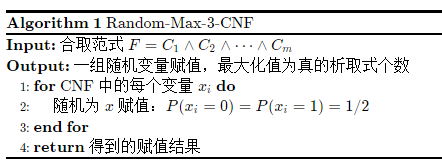DNF 满足性赋值：DNF 只需要一个合取子句为真，最终的结果就为真。DNF 满足性赋值要求输出能够使 $D_1, \cdots, D_m$ 之一满足的变量赋值。其实 SAT 问题既可以针对 CNF，也可以针对 DNF，我这里沿用老师在课堂上的讲法，将 SAT 问题专门用来分析 CNF 赋值问题，DNF 赋值问题用『DNF 满足性赋值』这个术语。

### 2-SAT 随机赋值算法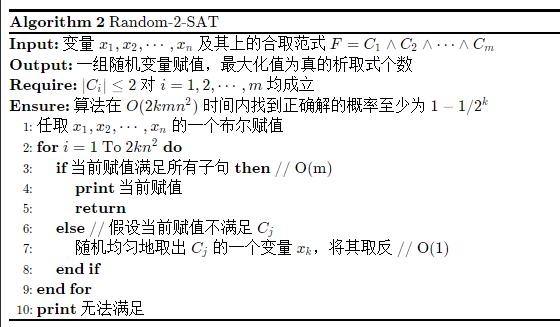1. 将第 7 步修改的过程抽象为随机游走，建立从『任意赋值』到『使范式为真的赋值』所需的步数与 $n$ 的数学关系。
2. 评价算法得到解得概率。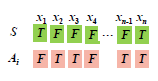1. $X_i=0$，即 $A_i$$S$ 对每一个变量的赋值都不同，则不管修改哪一个变量 $x_k$，都会变成和 $S$ 中对 $x_k$ 的赋值一样，则 $X_{i+1}=1$
2. $X_i \neq 0$，要进一步分情况讨论，我们不妨表示 $C_j=x_{j1} \lor x_{j2}$
1. 事件 $A$: 如果 $X_i$$x_{j1}, x_{j2}$ 的赋值与 $S$ 都不同，则 $X_{i+1}=X_i+1$
2. 事件 $B$: 如果 $X_i$$x_{j1}, x_{j2}$ 的赋值与 $S$ 只有一个不同，则 $X_{i+1}=\begin{cases} X_i+1, P = 1/2 \\ X_i-1, P = 1/2 \\ \end{cases}$

\begin{aligned} P(X_{i+1} = X_i +1) &= P(X_i=0)P(X_{i+1}=X_i+1|X_i=0) + P(X_i\neq 0)P(X_{i+1}=X_i+1|X_i\neq 0) \\ &= P(X_i=0) + P(X_i\neq 0) [P(A|X_i\neq 0) + P(B|X_i\neq 0) \frac{1}{2}] \\ &\ge P(X_i=0) + P(X_i\neq 0) [P(A|X_i\neq 0) \frac{1}{2} + P(B|X_i\neq 0) \frac{1}{2}] \\ &= P(X_i=0) + P(X_i\neq 0) \frac{1}{2} \\ &\ge P(X_i=0)\frac{1}{2} + P(X_i\neq 0) \frac{1}{2} \\ &= \frac{1}{2} \end{aligned}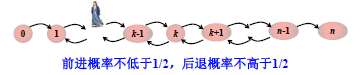$X_i \in [0, n]$，考虑最坏情况，$X_0=0$，即 $A_i$$S$ 完全不同，那么从状态 $0$ 到达状态 $n$ 需要多少步？给定步数，不能到达状态 $n$ 的概率又是多少？下面来进行分析。首先，为了简化问题，认为前进后退概率均为 $\frac{1}{2}$

$h_k$ 表示从状态 $k$ 到达状态 $n$ 需要的时间的随机变量，下面求解 $E(h_0)$

$h_k = \begin{cases} h_k+1, P = \frac{1}{2} \\ h_k-1, P = \frac{1}{2} \end{cases}$

$E(h_k) = 1+\frac{E(h_{k-1})}{2}+\frac{E(h_{k+1})}{2}$

\begin{aligned} & h_0 = 1+h_1 \\ & h_n=0 \end{aligned}

$E(h_0) = E(h_1+1) = E(h_1)+1=E(h_2)+3 = \cdots = 1+3+5+\dots+(2n-1)+0=n^2$

1. 计算所需运行次数均值
2. 利用 Markov、Chebyshev、Chernoff 界算一个给定运行次数之下仍然得不到解的尾概率
3. 重复算法多少遍，错误概率缩小，正确概率放大

$P(h_0 \ge 2n^2) \le \frac{E(h_0)}{2n^2} = \frac{1}{2}$

### 3-SAT 随机算法

3-SAT 如果还是用原来的 2-SAT 算法这个过程，那么算出来的从 $0$$n$ 的期望步数为 $E=(h_0) 2^{n+2}-3n-4$（前进概率 $1/3$，后退概率 $2/3$），而布尔赋值总共才只有 $2^n$ 种，必须改进。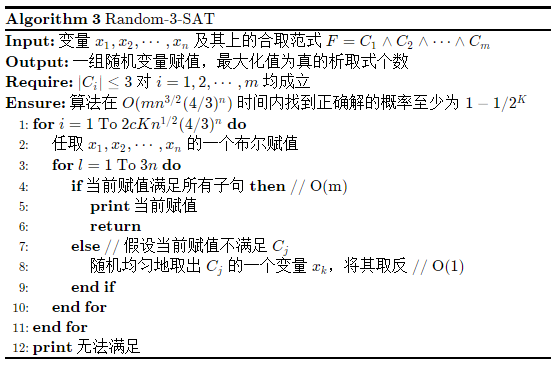$q$ 的证明过程比较复杂，一般人很难想到，可以查阅《概率与计算》。如果考试要证明这种概率，可以直接略过，先试着假设 $q$ 是一个常数，得到结论之后，仔细来计算这个 $q$，放缩真的是一门艺术，需要仔细斟酌。

### MAX-SAT 随机算法

#### Random-Sample 算法

Random-Sample 算法是一种随机抽样算法。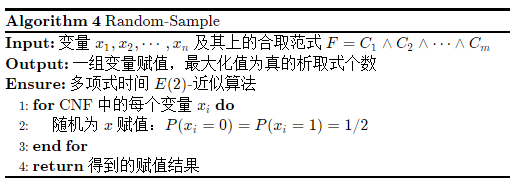$Y_i = \begin{cases} 1, 子句 C_i 被满足 \\ 0, 子句 C_i 未被满足 \end{cases}$

\begin{aligned} & P(C_i 未被满足) = \left(\frac{1}{2}\right)^{|C_i|} \le \frac{1}{2} \\ & P(C_i 被满足) = 1- P(C_i 未被满足) \ge \frac{1}{2} \end{aligned}

$E(Y_i) \ge \frac{1}{2}$

$E(Y) = \sum_{i=1}^mE(Y_i) \ge \frac{m}{2}$

$opt$ 为优化解满足的子句个数，我们得到：

$\frac{opt}{E(Y)} \le \frac{m}{E(Y)} \le 2$

#### Random-Round 算法

Random-Round 算法是一种随机舍入算法。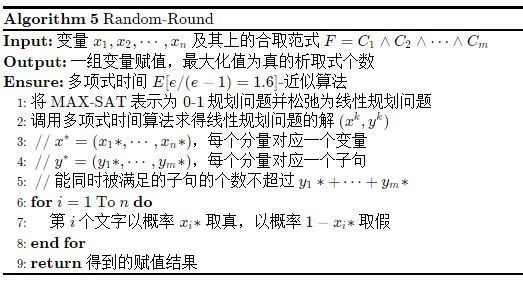$x_i = \begin{cases} 1, 第 i 个变量取真 \\ 0, 第 i 个变量取假 \end{cases}$

$y_j = \begin{cases} 1, 子句 C_j 被满足 \\ 0, 子句 C_j 未被满足 \end{cases}$

MAX-SAT 表示成 0-1 规划问题，就是要：

1. 最大化满足的子句个数

$\max y_1 + y_2 + \cdots + y_m$

2. 将子句满足与否用数学式子表示

$s.t. \sum_{i\in C_j^+} x_j + \sum_{i\in C_j^-}(1-x_j) \ge y_j, \quad 1 \le j \le m$

3. 值域要求

$x_i \in \{0, 1\}, \quad 1 \le i \le n$

$y_j \in \{0, 1\}, \quad 1 \le j \le m$

$E(Y) = \sum_{i=1}^mE(Y_j) \ge \sum_{j=1}^m (1-1/e)y_j^*$

$\frac{opt}{E(Y)} \le \frac{y_1*+\cdots+y_m*}{E(Y)} \le \frac{e}{e-1} \approx 1.6$

\begin{aligned} P(C_j不被满足) &= \prod_{i \in C_i^+}(1-x_i^*)\prod_{i \in C_i^-}x_i^* \\ &= \left( \left( \prod_{i \in C_i^+}(1-x_i^*)\prod_{i \in C_i^-}x_i^* \right)^{1/|C_j|} \right)^{|C_j|} \\ &\le \left( \frac{\sum_{i \in C_i^+}(1-x_i^*)\sum_{i \in C_i^-}x_i^*}{|C_j|} \right)^{|C_j|} \\ &= \left(1- \frac{\sum_{i \in C_i^+}x_i^*\sum_{i \in C_i^-}(1-x_i^*)}{|C_j|} \right)^{|C_j|} \\ &\le \left( 1-\frac{y_j^*}{|C_j|} \right)^{|C_j|} \end{aligned}

$P(C_j 被满足) \ge 1-\left( 1-\frac{y_j^*}{|C_j|} \right)^{|C_j|}$

$1-(1-r/k)^k \ge [1-(1-1/k)^k]r$$r\in[0, 1]$ 和整数 $k$ 成立。

\begin{aligned} P(C_j 被满足) &\ge 1-\left( 1-\frac{y_j^*}{|C_j|} \right)^{|C_j|} \\ & \ge \left[ 1- (1-1/|C_j|)^{|C_j|} \right] y_j^* \\ &\ge (1-1/e)y^*_j \end{aligned}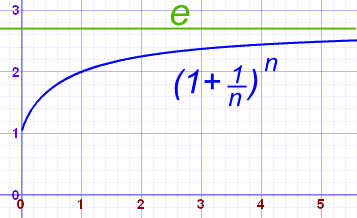#### Random-Mix 算法

Random-Mix 算法是一个混合随机算法。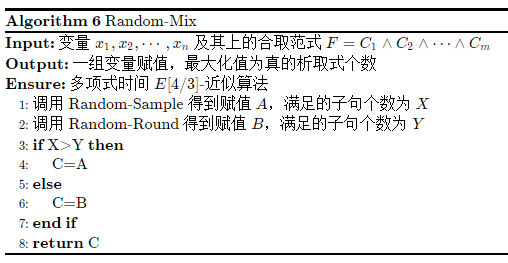\begin{aligned} E(Z) &\ge \frac{1}{2}E(X+Y) \\ &= \sum_{i=1}^mE(X_i) + \sum_{i=1}^mE(Y_i) \\ &= \sum_{i=1}^m\left[ E(X_i) + E(Y_i) \right] \\ \end{aligned}

$E(X_i) = 1\cdot P(C_i=1) = 1 - \frac{1}{2^{|C_i|}} \ge \left( 1 - \frac{1}{2^{|C_i|}} \right) y_i^*$

$E(Y_i) \ge \left[ 1- (1-1/|C_j|)^{|C_j|} \right] y_i^*$

\begin{aligned} E(X_i) + E(Y_i) &\ge \left( 1 - \frac{1}{2^{|C_i|}} \right) y_i^* + \left[ 1- (1-1/|C_i|)^{|C_i|} \right] y_i^* \\ &= \left\{ \left( 1 - \frac{1}{2^{|C_i|}} \right) + \left[ 1- (1-1/|C_i|)^{|C_i|} \right] \right\}y_i^* \\ &\ge \frac{3}{2}y_i^* \end{aligned}

$\frac{opt}{E(Z)} \le \frac{4}{3}$

#### 去随机化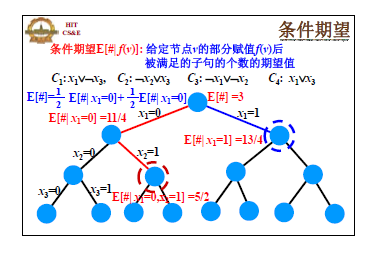##### DetAssign 算法

$g(u) = \frac{1}{2}g(v) + \frac{1}{2}g(w)$

\begin{aligned} Random-Sample 算法的平均情况 &= E(\#) \\ &\le g(u_0) \le g(u_1) \cdots \le g(u_n) \\ &= DetAssign 算法返回的 x_1, \cdots, x_n 赋值满足的子句个数 sat \end{aligned}

##### DetRound 算法

$g(u) = g(v)(1-x_i^*) + g(w)x_i^*$

### DNF 满足性赋值随机算法

DNF 是析取范式，要求一个合取子句满足即可，我们要求解的是能够使 $D_1, \cdots, D_m$ 之一被满足的可行的对 $x_1, \cdots, x_n$ 的赋值个数 $c(F)$

#### 朴素算法

$P[|X/N - c(F)/2^n| > \epsilon c(F)/2^n] \le \delta \\$

$X_i=1$ 表示第 $i$ 次抽样的随机样本满足某个合取式，由于 $\mu = E(X/N) =E(X)/N=\sum_{i=1}^NE(X_i)/N=c(F)/2^n$，所以：

$P[|X/N - \mu| > \epsilon \mu] \le 2\exp{-\frac{\mu \epsilon^2}{3}} \le \delta$

\begin{aligned} \mu &\ge -3\ln \frac{\delta}{2} \end{aligned}

$P[X-\mu'>\epsilon \mu'] \le 2 \exp{-\frac{\mu' \epsilon^2}{3}} \le \delta$

\begin{aligned} \mu' &\ge -\frac{3}{\epsilon^2}\ln \frac{\delta}{2} \\ N &\ge 3\cdot 2^n\ln(2/\delta)/\epsilon^2 c(F) \end{aligned}

#### Buboly-Karp 算法

$|U| = \sum_{i=1}^m |SC_m|$

\begin{aligned} P[取中 (i, a)] &= P(在 SC_i 中抽取) P(取中 (i, a)|在 SC_i 中抽取) \\ &= |SC_i|/|U| \cdots \frac{1}{|SC_i|} \\ &= \frac{1}{|U|} \end{aligned}

## 集合平衡配置问题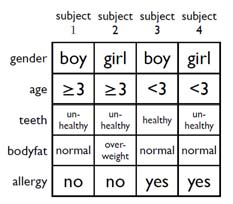$\begin{pmatrix} a_{11} & a_{12} & \cdots & a_{1m} \\ a_{21} & a_{22} & \cdots & a_{2m} \\ \vdots & \vdots & \ddots & \vdots \\ a_{n1} & a_{n2} & \cdots & a_{nm} \end{pmatrix}_{n\times m} \begin{pmatrix} x_1 \\ x_2 \\ \vdots \\ x_m \end{pmatrix}_{m \times 1} = \begin{pmatrix} c_1 \\ c_2 \\ \vdots \\ c_n \end{pmatrix}_{n \times 1}$

$P[|C|_\infty \ge \sqrt{12m\ln n}] < \frac{2}{n}$

\begin{aligned} P(|C|_\infty > t) &= P\left(\cup_{i=1}^n\left|\sum_{j=1}^{m}a_{ij}x_j\right| > t\right) \\ & \le \sum_{i=1}^n P\left(\left|\sum_{j=1}^{m}a_{ij}x_j\right| > t\right) \tag{1} \end{aligned}

$Y_j = \begin{cases} 1, \quad P(Y_j=1)=\frac{1}{2} \\ 0, \quad P(Y_j=0)=\frac{1}{2} \end{cases}$

\begin{aligned} P\left(\left|\sum_{j=1}^{m}a_{ij}x_j\right| > t\right) &\le P\left[\left(Y<\frac{m}{2}-\frac{t}{2}\right) \lor \left(Y>\frac{m}{2}+\frac{t}{2}\right)\right] \\ &= P\left(|Y-\mu| > \frac{t}{2}\right) \\ &= P\left(|Y-\mu| > \delta \mu\right), \quad \delta=\frac{t}{m} \\ &\le 2\exp{-\frac{\mu \delta^2}{3}} \\ &= 2\exp{-\frac{t^2}{6m}}, \quad 取 t = \sqrt{12m\ln n} \\ &= \frac{2}{n^2} \end{aligned}

$P(|C|_\infty > \sqrt{12m\ln n}) \le n \times \frac{2}{n^2} = \frac{2}{n}$

### 去随机化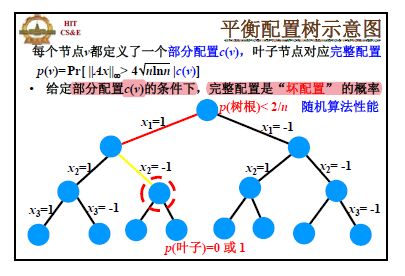$p(u) = p(v)P(x_i=1) + p(w)P(x_i=0)$

\begin{aligned} q(u) &= \min \left( 1, \sum_{j}P\left[|C_j| > 4\sqrt{n \ln n} | c(u)\right] \right) \\ &\ge P\left[\lor_{j} \left( |C_j| > 4\sqrt{n \ln n} | c(u) \right)\right] \\ &= P\left[|C_j|_\infty > 4\sqrt{n \ln n} | c(u)\right] \\ &= p(u) \end{aligned}

$q$ 满足三个性质：

1. $q(u) = 0 \Rightarrow p(u) = 0$

2. $q(叶子) = 0或1$，一旦具体的配置方案确定，要么满足 $|C|_\infty \ge 4\sqrt{n\ln n}$，要么不满足

3. $q(树根) \le 2/n$

根据之前的结论可以得到。

## 最大割问题

$Y_j = \begin{cases} 1, \quad 边 j 的两个顶点分别在 V 和 S_i -V 中, p = \frac{1}{2} \\ 0, \quad 边 j 的两个顶点同时在 V 和 S_i - V 中, p = \frac{1}{2} \end{cases}$

$p(c_i \ge \frac{m}{2}) \le 1$

\begin{aligned} \frac{m}{2} &= E(c_i) \\ &= \sum_{k}k\cdot P(c_i=k) \\ &= \sum_{k < m/2} k \cdot P(c_i < \frac{m}{2}) + \sum_{k \ge m/2} k \cdot P(c_i\ge \frac{m}{2}) \\ &\le (m/2-1)(1-p) + mp \end{aligned}

\begin{aligned} P[X \ge 2E(X)] &\le \frac{1}{2} \\ P[X \ge m+2] &\le \frac{1}{2} \\ \end{aligned}

1. 《概率与计算》
2. 《随机算法》